# Switch statement in Java

Switch statement in Java is a statement used to check the value of a variable, which can have different values, depending on the value of this variable we can define a different set of statements.

For example, I have n variable that can be valued at 1,2,3. I want that in case the value of this n variable is 1, I will print the word “Khanh”, the value of 2 will print the word “Huong Dan Java”, the value of 3 will print “Lap Trinh Java “, using a switch statement, I will write the following code:

Here, we use the case label to handle each different value of n variable. The declared value for each case must be COMPILE TIME CONSTANTS. This means that when compiling source code, the value of each case must be determined, not waiting until the program runs, execute to this switch code, the value of each case will then be determined.

To execute only the code for each value of n variable, we must use the break keyword so that after executing the code, our program will exit the switch statement.

As you can see, we also have a default label, this label is used to handle cases where the value of this n variable is not in the case where the switch statement has been processed. In a switch statement, we may not need to declare the default label, if it is declared then it is not necessary to declare on a certain order!

The result when executing the above code will look like this: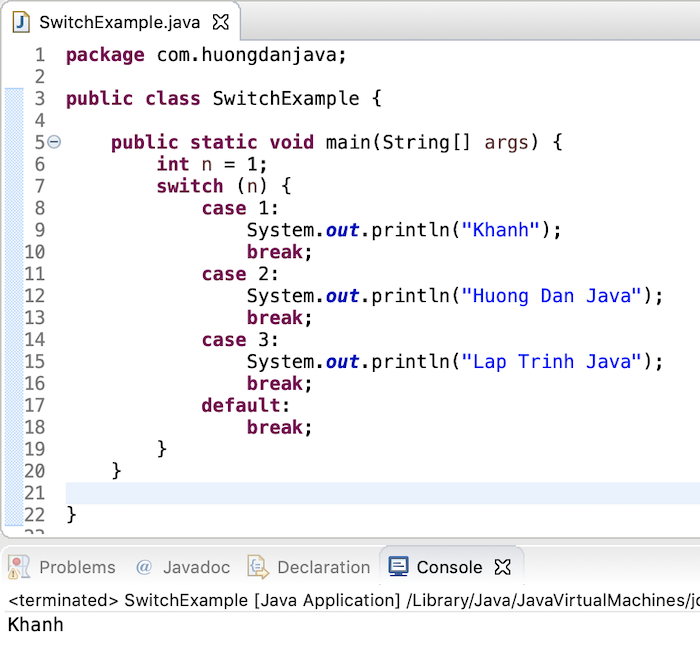As you can see, because the value of n variable, in this case, is 1, the case for value 1 will be executed and print “Khanh”. After printing the text, to the break statement, our program will exit the switch statement.

Before Java 7, you can pass the variable of type char, byte, short, int or their wrapper Character, Byte, Short, Integer and Enum in a switch statement. From Java 7 onwards, the switch statement also supports String data type.

Result: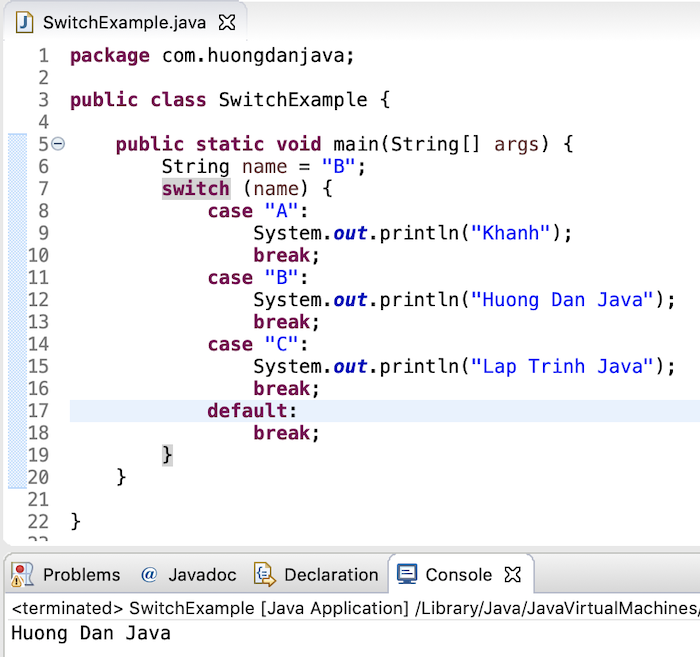You can pass into the switch statement an expression as long as the return value of this expression is the allowable type of the switch statement.

Result:### Switch from Java 12

When working with a switch statement, you can encounter the following problems:

• The first problem is that we forget to declare the break statement: this means that our code will not exit the switch statement after matching the first case but will continue the next case, for example:

Result: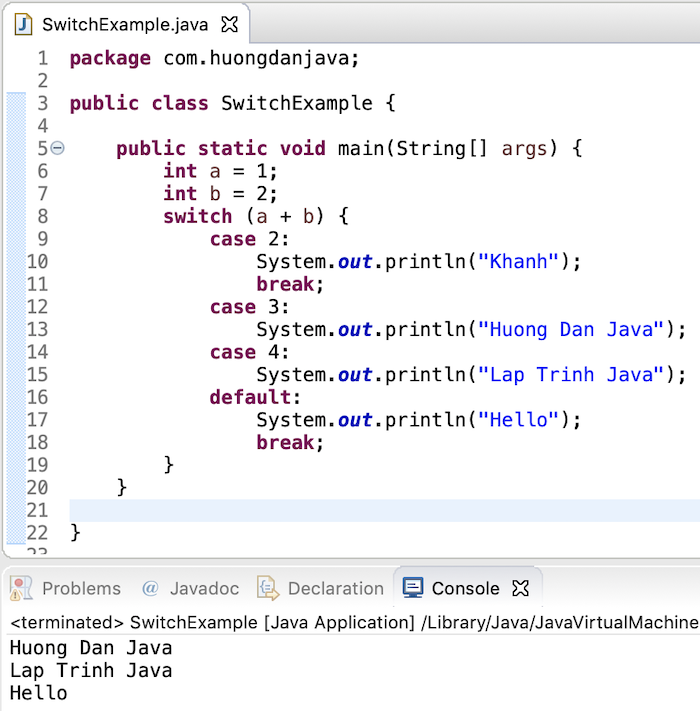• The second problem is if we want in case the value of the variable with different values a and b, we will handle the same code. We will write the following code:

Or:

These ways of writing are inconvenient because of duplicating the code or we sometimes can’t control it, where should we put a break statement?

• The third problem is that if you want to use a switch statement to determine the value of a variable, you must write the following:

To solve these problems, from Java 12, you can write a switch statement with the following improvements:

• For the first problem, Java 12 now supports us on how to write a switch statement like lambda expression, for example. as:

Result: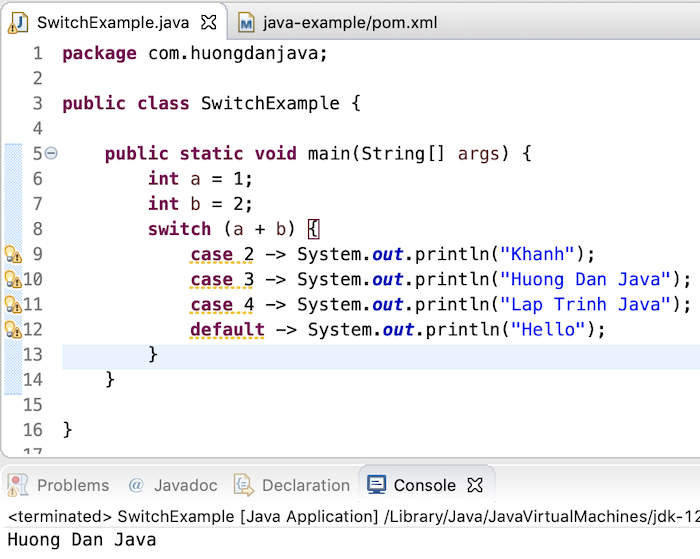• For the second problem, you can rewrite the switch statement as follows:

Result: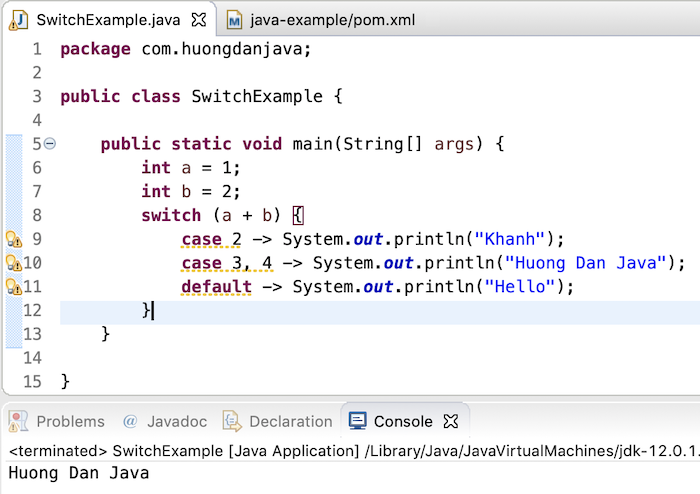• As for the third problem, now Java 12 has supported us to return value with a switch expression as follows:

Result:### Switch from Java 13

If you want to add code to handle business logic in each case of the switch expression, then you can upgrade to Java 13 and add the code to use the yield keyword to return the value you want. The example is as follows:

Result: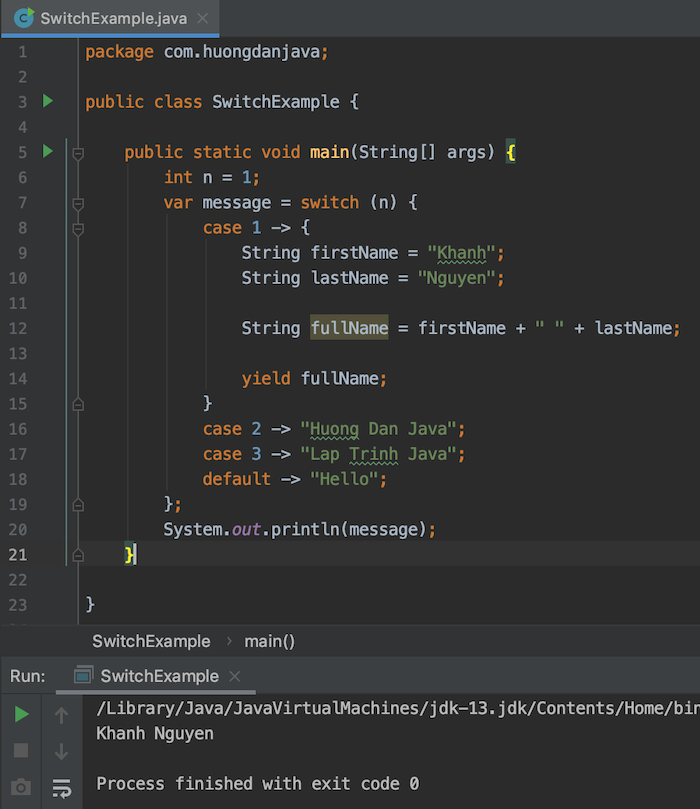### Switch from Java 17

Since Java 17, the switch supports pattern matching similar to the instanceof operator from Java 14.

For example, I have an interface with two implementations as follows:

To check and print the implementation of a Shape object, we would normally write code like this:

From Java 17, you can write briefly with the switch as follows:

In this case, you must declare the default case! Because the shape object may not be in the implementations that we declared in the cases.